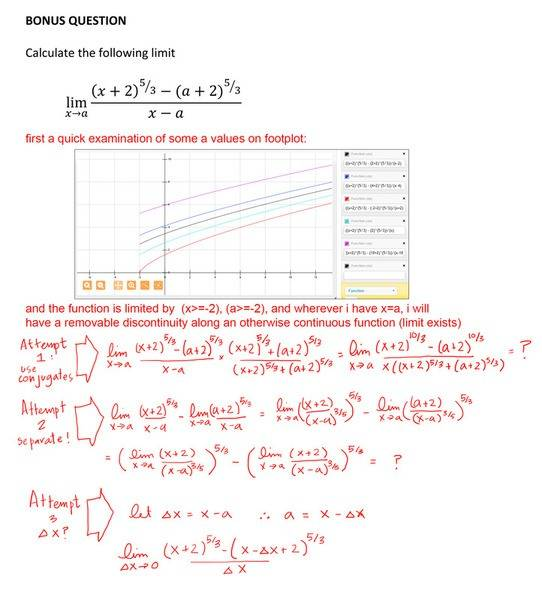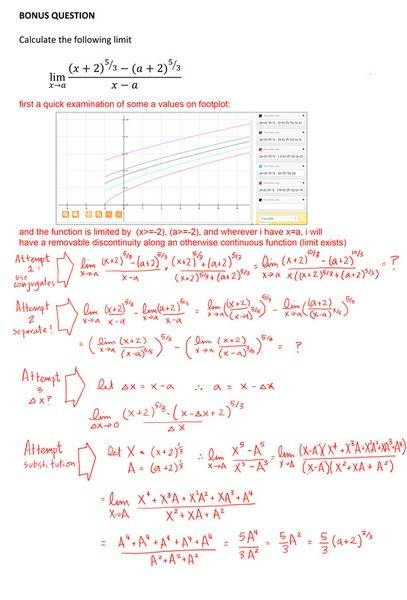# Trying to figure out a limit as x-->a

• fishspawned
In summary, the limit x->a of [(x+2)^5/3 - (a+2)^5/3] / (x-a) is homework equation. The Attempt at a Solution mentions that differentiation is the shortest way. The restriction is due to the question is apparently brought up before any derivatives are introduced. So apparently this is do-able without using straight up differentiation. The 1/3 is killing me. and i have no idea how to approach it.

## Homework Statement

the limit x->a of [(x+2)^5/3 - (a+2)^5/3] / (x-a)

limit laws

## The Attempt at a Solution[/B]

Does the formula in the third attempt remind you on something?

it looks like I'm trying to get a derivative. I am trying to do this without working using any derivatives and for some reason the power of 5/3 is completely messing with my head and i do not know how to proceed. i am still getting a 0/0 situation.

Well, differentiation seems to be the shortest way. Otherwise you probably will have to follow the paths the differentiating formulas are proven. The power ##\frac{1}{3}## is the difficulty here, for you cannot expand it easily. Why do you want to restrict yourself, once you already have the formula for the first derivative?

The restriction is due to the question is apparently brought up before any derivatives are introduced. So apparently this is do-able without using straight up differentiation. Yes, the 1/3 is killing me. and i have no idea how to approach it.

I've just looked up how ##\frac{d}{dx} x^{\alpha}=\alpha x^{\alpha-1}## is proven for non-integer values ##\alpha##.
It's done by the chain rule and ##x^\alpha = \exp(\alpha \ln x)##. Perhaps this might help and you may use properties of the exponential function. Another substitution ##y=x+2## should decrease writing work.

Set ##X=(x+2)^{1/3}## ##A=(a+2)^{1/3}## then the it becomes ##\lim_{X\rightarrow A}{\frac{X^5-A^5}{X^3-A^3}}## . Hope I am right here and this helps.

•fresh_42
DELTA:
this seems to take care of it - provided you know how to expand a sum of powers to a high level - had to look it up to be honest:

so revised sheet:•Delta2 and fresh_42
fishspawned said:
DELTA:
this seems to take care of it - provided you know how to expand a sum of powers to a high level - had to look it up to be honest:

so revised sheet:Correct result.